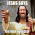## Thursday, January 15, 2015

### NFL Picks Contest- Divisional Playoff Results

Here are the Divisional Playoff results and standings in the NFL Picks Contest. Only two weeks left. Will cokeboy hold on or will OneAndDone pull ahead? And two-time defending champion MOJO still has a shot at a three-peat!

Here are the current standings;

cokeboy99- 27-(8)+21+34+16+13+20+38+16+51+43+13+32+16+20+39+24+14+24 = 453 pts.
OneAndDone- 27+17+17+34+41+5+31+38+16+24+17+30+32+16+18+27+20+14+24 = 448 pts.
MOJO-  27+17+17+29+16 +24+32+37+16+34+17+30+32+16+18+12+20+21+24 = 439 pts.
Vegas Vic- 27+17+21+29+16+5+32-(3)+20+31+17+29+32+16+17+39+24+14+14 = 397 pts.

lightning36- 27+17+17+29+16+24+31+24+20+34-(15)+30+32-(31)+18+27+43+14+24 = 384 pts.
Music City Momma- 13+17+21+29+16-(7)+32+38-(3)+31+17+30+32+17+17+39+43-(13)+14 = 383 pts.
TwoMinuteWarning- (3)+17+37+29+16-(7)+35+37+20+24-(17)+19+32+17+18+39+24+14+24 = 375 pts. Captain Crunch- 27+17+17+29+16+5+35+38+24+24-(17)+30+32+34+17+11+20-(13)+24 = 370 pts.

Grange95- (3)+17+21+29+16+24+11+20+16+0+43+13+62+34-(8)+16+20+26-(11) = 346 pts.
mrben09- 27+17+17+29+16+24+31+37-3+24-(15)+30+32+16+18+11+16-(13)+24 = 338 pts.
angerisagift- (16)+41+37-(27)+18+41+31+24+13+24-(2)-(3)+13+13-(7)+45-(10)+21+0 = 256 pts.
Coach- 27+17+21+29+20+24+20-(3)+16-(7)-(20)+14+11+16+26+11+20+14-(11) = 245 pts.

Divisional scores

angerisagift- No Pick = 0 pts.
MOJO- Seattle (W 31-17)= 24 pts.
Vegas Vic- New England (W 35-31)= 14 pts.
Captain Crunch- Seattle (W 31-17)= 24 pts.

OneAndDone- Seattle (W 31-17)= 24 pts.
mrben09- Seattle (W 31-17)= 24 pts.
Coach- Denver (L 13-24)= (11) pts.
lightning36- Seattle (W 31-17)= 24 pts.

Music City Momma- New England (W 35-31)= 14 pts.
TwoMinuteWarning- Seattle (W 31-17)= 24 pts.
Grange95- Denver (L 13-24) = (11) pts.
cokeboy99- Seattle (W 31-17)= 24 pts.

Thanks for playing.

Conference Championship picks are due by Saturday at 6pm (CST). All games are eligible.

Good luck!

1.2.